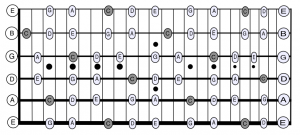Guitar Lessons Las Vegas: Learn Guitar With Vince Lauria! Call 702.626.9155

# How to Play, Understand & Figure Out All Scales-Part VIII

Nov 22, 2017 Comments are off

Using the C major scale as the foundation for all scales. Now learn how to alter the C major scale to create the C Major Pentatonic Scale. I will refer to it as the C Ionian Pentatonic Scale. The diagram below is for guitar but the note relationship or distances remains the same for all instruments.

Memorize and play the C Ionian Pentatonic Scale. Just omit the F or fourth and the B or seventh scale notes. Also called the tritone.

ALL scales repeat the same pattern up and down in tone. C major scale: C, D, E, F, G, A, B and C. Numbers are given to correspond to the major scale degrees or positions.

C Major Scale: C=1/Root, D=2nd, E=3rd, F=4th, G=5th, A=6th, B=7th, C=8th/octave.

C Ionian Pentatonic Scale: C=1/Root, D=2nd, E=3rd, G=5th, A=6th.

Memorize the C Ionian Pentatonic Scale note names and number formula.

C Ionian Pentatonic – note names= C, D, E, F, G, A, and C

C Ionian Pentatonic – number formula = 1/R, 2nd, 3rd, 5th, 6th, and root/8.

C, D, E, G, A, C

1, 2, 3, 5, 6, 8/1.

Using the note name chart below – play the C ionian pentatonic scale starting from low thick E string 1st fret up neck, then back down neck. Repeat this same process for each string. Remember to say note names out loud as you play each note up and then down in tone. Repeat this process for all 6 strings: Low E, then A, D, G, B and thin E string. The underlined fret number indicates dot positions:

open   1        3,      5,        7,      9,           12,          15,     17     19,    21,       24Now play and say out loud the C ionian pentatonic scale for each note up and back each string. Play the C note twice ascending and descending to memorize root note positions.

E, G, A, C, D, E – E, D, C, A, G, E

this is C Ionian Pentatonic Scale starting on the low thick E string E to E octave.

A, C, D, E, G, A – A, G, E, D, C, A

this is C Ionian Pentatonic Scale starting on A to A octave.

D, E, G, A, C, D – D, C, A, G, E, D

this is C Ionian Pentatonic Scale starting on D to D octave.

G, A, B, C, D, E, F, G – G, F, E, D, C, B, A, G

this is C Ionian Pentatonic Scale starting on G to G octave.

(B) C, D, E, G, A, C – C,  A, G, E, D, C,

this is C Ionian Pentatonic Scale starting on the B string first fret to C octave 13th fret.

E, G, A, C, D, E – E, D, C, A, G, E

this is C Ionian Pentatonic Scale starting on the high thin E string E to E octave.

The note to note intervals are the same. This applies to any string, octave, position, instrument or Key. Repeat this same process for bass, piano and any other instrument.

All materials for personal use only.

Vince Lauria Sun and Earth Music